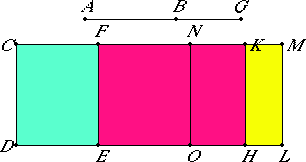# Proposition 100

The square on a minor straight line applied to a rational straight line produces as breadth a fourth apotome.

Let AB be a minor and CD a rational straight line, and to the rational straight line CD let CE be applied equal to the square on AB and producing CF as breadth.

I say that CF is a fourth apotome.X.76

Let BG be the annex to AB. Then AG and GB are straight lines incommensurable in square which make the sum of the squares on AG and GB rational, but twice the rectangle AG by GB medial.

To CD apply CH, equal to the square on AG, producing CK as breadth, and KL, equal to the square on BG, producing KM as breadth. Then the whole CL equals the sum of the squares on AG and GB.

And the sum of the squares on AG and GB is rational, therefore CL is also rational.

X.20

And it is applied to the rational straight line CD producing CM as breadth, therefore CM is also rational and commensurable in length with CD.

II.7

Since the whole CL equals the sum of the squares on AG and GB, and, in these, CE equals the square on AB, therefore the remainder FL equals twice the rectangle AG by GB.

Bisect FM at the point N, and draw NO through N parallel to either of the straight lines CD or ML. Then each of the rectangles FO and NL equals the rectangle AG by GB.

And, since twice the rectangle AG by GB is medial and equals FL, therefore FL is also medial.

X.22

And it is applied to the rational straight line FE producing FM as breadth, therefore FM is rational and incommensurable in length with CD.

Since the sum of the squares on AG and GB is rational, while twice the rectangle AG by GB is medial, therefore the sum of the squares on AG and GB is incommensurable with twice the rectangle AG by GB.

But CL equals the sum of the squares on AG and GB, and FL equals twice the rectangle AG by GB, therefore CL is incommensurable with FL.

But CL is to FL as CM is to MF, therefore CM is incommensurable in length with MF.

X.73

And both are rational, therefore CM and MF are rational straight lines commensurable in square only. Therefore CF is an apotome.

I say that it is also a fourth apotome.

Since AG and GB are incommensurable in square, therefore the square on AG is also incommensurable with the square on GB. And CH equals the square on AG, and KL equal to the square on GB, therefore CH is incommensurable with KL.

But CH is to KL as CK is to KM, therefore CK is incommensurable in length with KM.

Since the rectangle AG by GB is a mean proportional between the squares on AG and GB the square on AG equals CH, the square on GB equals KL, and the rectangle AG by GB equals NL, therefore NL is a mean proportional between CH and KL. Therefore CH is to NL as NL is to KL.

But CH is to NL as CK is to NM, and NL is to KL as NM is to KM, therefore CK is to MN as MN is to KM.

VI.17

Therefore the rectangle CK by KM equals the square on MN, that is, to the fourth part of the square on FM.

X.18

Since CM and MF are two unequal straight lines, and the rectangle CK by KM, equal to the fourth part of the square on MF and deficient by a square figure, has been applied to CM and divides it into incommensurable parts, therefore the square on CM is greater than the square on MF by the square on a straight line incommensurable with CM.

X.Def.III.4

And the whole CM is commensurable in length with the rational straight line CD set out, therefore CF is a fourth apotome.

Therefore, the square on a minor straight line applied to a rational straight line produces as breadth a fourth apotome.

Q.E.D.

## Guide

This proposition is used in X.111.